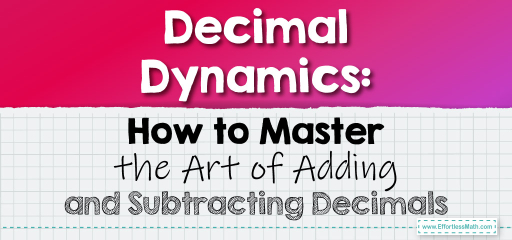# Decimal Dynamics: How to Master the Art of Adding and Subtracting Decimals

Decimals represent parts of whole numbers and are essential in various real-world scenarios, from measuring distances to handling money. Adding and subtracting decimals might seem tricky at first, but with a systematic approach, it becomes straightforward. Let's explore the steps and strategies to effectively add and subtract decimals.

Key Insight:

When adding or subtracting decimals, it's crucial to line up the decimal points. This ensures that each place value is correctly aligned.Add $$23.45$$ and $$7.689$$.

Solution Process:

1. Write the numbers vertically, aligning the decimal points.

2. Start adding from the rightmost column (smallest place value).

3. Carry over any value greater than $$9$$ to the next column.

The sum is $$31.139$$.

The Absolute Best Book for 5th Grade Students

### Example 2: Subtracting Decimals

Subtract $$15.82$$ from $$20.5$$.

Solution Process:

1. Write the numbers vertically, aligning the decimal points.

2. If necessary, add zeros to make the numbers have the same number of decimal places.

3. Start subtracting from the rightmost column.

4. Borrow from the next column if needed.

The difference is $$4.68$$.

Adding and subtracting decimals is a fundamental skill in mathematics. The key is to ensure that the decimal points are aligned, allowing for accurate calculations. Whether you’re calculating distances, handling money, or measuring quantities, understanding how to add and subtract decimals is essential. With practice, you’ll find that working with decimals becomes second nature, enabling you to tackle a wide range of mathematical and real-world challenges with confidence!

### Practice Questions:

1. Add $$12.34$$ and $$5.678$$.

2. Subtract $$9.01$$ from $$10.5$$.

3. Add $$45.6$$, $$23.45$$, and $$7.89$$.

4. Subtract $$32.8$$ from $$50$$.

5. Add $$0.123$$, $$4.56$$, and $$7.89$$.

A Perfect Book for Grade 5 Math Word Problems!

1. $$18.018$$

2. $$1.49$$

3. $$76.94$$

4. $$17.2$$

5. $$12.563$$

The Best Math Books for Elementary Students

### What people say about "Decimal Dynamics: How to Master the Art of Adding and Subtracting Decimals - Effortless Math: We Help Students Learn to LOVE Mathematics"?

No one replied yet.

X
51% OFF

Limited time only!

Save Over 51%

SAVE $15 It was$29.99 now it is \$14.99